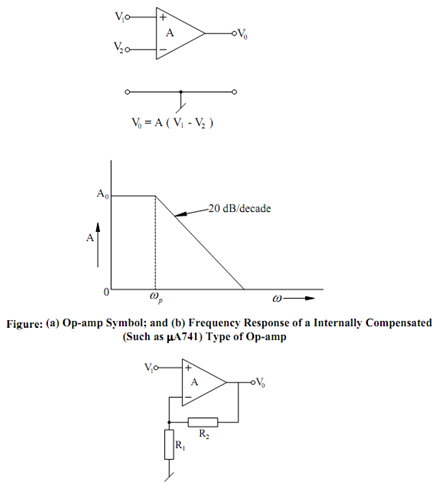## Effect of Op-amp Pole Assignment Help

Assignment Help: >> Basic Analog Circuits using Ideal Op-amps - Effect of Op-amp Pole

Effect of Op-amp Pole and the Techniques of Active Compensation:

Two dominant non-idealities which limit the operational frequency range of op-amp circuits (designed on the basis of ideal hypothesis of infinite gain) beyond a few tens of kHz are the slew-rate and frequency-dependent finite open loop given A of the op-amps. A typical value for the slew rate, taking the example of 741 type IC op-amp, is around 1 volt/μsec. Alternatively, the open loop gain A designed to have a dominant pole, to guarantee stable operation under every feedback conditions exhibits the frequency response as illustrated in Figure. It so called 'one pole roll-off' characteristic may be represented by a first order transfer function.

A =   A0 ωρ /S + ωρ

For frequency range given by f > > fρ , A may be approximated by

A ≅  A0 ωρ /SFigure: Op-amp Configured as a Non-inverting Amplifier

 Active-compensated Non-inverting Amplifier Magnitude Error Measurement of the Gain Bandwidth-Product of the Op-amp Realising Some Useful Functional Circuits